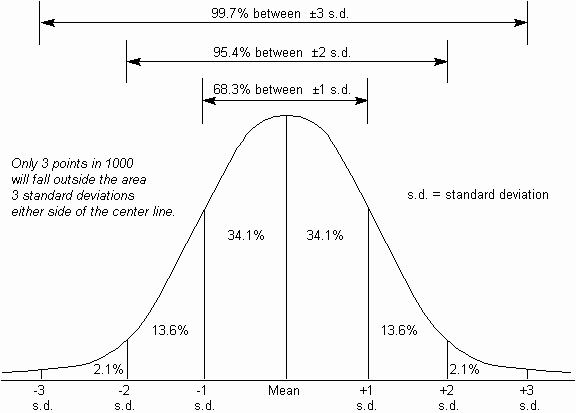The Psychology of Quality and More

# Standard Deviation

The standard deviation is a number which is calculated using a simple mathematical trick (calculating the square root of the average of squares) to find an 'average' number for the distance of the majority of measures from the mean.

The standard deviation is usually a preferable method of measuring spread, as opposed to the simpler 'Range' calculation, as it takes account of all measurements.

The Greek letter, sigma, (s) is often used to signify standard deviation.

It is of particular value when used with the Normal distribution, where known proportions of the measurements fall within one, two and three standard deviations of the mean, as below.### You can buy books here

And the big
paperback book